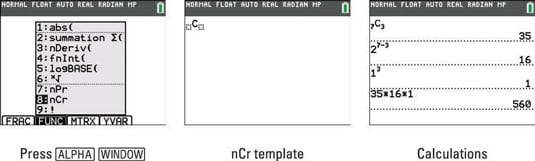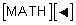##### TI-84 Plus CE Graphing Calculator For Dummies, 3rd EditionIn math class, you may be asked to expand binomials, and your TI-84 Plus calculator can help. This isn’t too bad if the binomial is (2x+1)2 = (2x+1)(2x+1) = 4x2 + 4x + 1. That's easy. What if you were asked to find the fourth term in the binomial expansion of (2x+1)7? Now that is more difficult.

The general term of a binomial expansion of (a+b)n is given by the formula: (nCr)(a)n-r(b)r. To find the fourth term of (2x+1)7, you need to identify the variables in the problem:

• a: First term in the binomial, a = 2x.

• b: Second term in the binomial, b = 1.

• n: Power of the binomial, n = 7.

• r: Number of the term, but r starts counting at 0. This is the tricky variable to figure out. Think of this as one less than the number of the term you want to find. Since you want the fourth term, r = 3.

Plugging into your formula: (nCr)(a)n-r(b)r = (7C3) (2x)7-3(1)3.

1. Press [ALPHA][WINDOW] to access the shortcut menu.

See the first screen.2. Press  to choose the nCr template.

See the first screen.

On the TI-84 Plus, pressto access the probability menu where you will find the permutations and combinations commands. Using the TI-84 Plus, you must enter n, insert the command, and then enter r.

3. Enter n in the first blank and r in the second blank.

Alternatively, you could enter n first and then insert the template.

4. Press [ENTER] to evaluate the combination.

5. Use your calculator to evaluate the other numbers in the formula, then multiply them all together to get the value of the coefficient of the fourth term.

See the last screen. The fourth term of the expansion of (2x+1)7 is 560x4.